Chapter 3 - Tangents, Normals, and Slopes of CurvesCustom SearchCHAPTER 3 TANGENTS, NORMALS, AND SLOPES OF CURVES LEARNING OBJECTIVES Upon completion of this chapter, you should be able to do the following: 1. Find the slope and equation of the tangent line for a standard parabola and for other curves at a given point. 2. Find equations of the tangent line and the normal line and lengths of the tangent and the normal between a point on a curve and the X axis for various curves. 3. Apply parametric equations to motion in a straight line and in a circle; and also, find equations and lengths of tangents and normals of curves using parametric equations. INTRODUCTION In chapter 1, the notation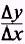was introduced to represent the slope of a line. The straight line discussed had a constant slope and the symbol Ay was defined as (y2 - y1) and Ax was defined as (x2 - x1). In this chapter we will discuss the slope of curves at specific points on the curves. We will do this with as little calculus as possible, but our discussion will be directed toward the study of calculus. SLOPE OF A CURVE AT A POINT In figure 3-1 the slope of the curve is represented at two different places by. The value of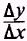Ay on the lower part of the curve is extremely close to the actual slope at P1 because P1 lies on a nearly straight portion of the curve. The value of the slope at P2 is less accurate than the slope near P1 because P2 lies on a portion of the curve that has more curvature than the portion of the curve near P1. To obtain an accurate measure of the slope of the curve at each point, as small a portion of the curve as possible should be used. When the curve is nearly a straight line, a very small error will occur when you are finding the slope, regardless of the value of the incrementsy andx. If the curvature is great and large increments are used when you are finding the slope of a curve, the error will become very large. Thus, the error can be reduced to as small an amount as you want provided you choose your increments to be sufficiently small. Whenever the slope of a curve at a given point is desired, the incrementsy andx should be extremely small. Consequently, the arc of the curve can be replaced by a straight line, which determines the slope of the line tangent to the curve at that point. You must understand that when we speak of the line tangent to a curve at a specific point, we are really considering the secant line, which cuts a curve in at least two points. Refer to figure 3-2. As point P2 moves closer to point P1, the slope of the secant line varies by smaller and smaller amounts and causes the secant line between P1 and P2 to approach P1 . P1 is then extended to form the line tangent to the curve at that specific point. If we allow to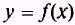represent the equation of a curve, then ~ is the slope of the line tangent to the curve at P(x,y).Figure 3-1.-Curve with increments Ay and Ax.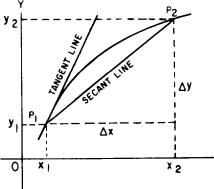Figure 3-2.-Curve with secant line and tangent line. The direction of a curve is defined as the direction of the tangent line at any point on the curve. Letequal the inclination of the tangent line; then the slope equals tanandis the slope of the curve at any point P(x,y). The angle, is the inclination of the tangent line at P, in figure 3-3. This angle is acute and the value of tan 0, is posŁitive. Hence, the slope of the tangent line is positive at point P1. The angle2 is an obtuse angle, tan2 is negative, and the slope of the tangent line at point P2 is negative. All lines leaning to the right have positive slopes, and all lines leaning to the left have negative slopes. At point P3 the tangent line to the curve is horizontal andequals 0. This means that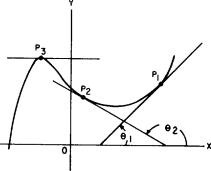Figure 3-3.-Curve with tangent lines.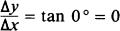The fact that the slope of a curve is zero when the tangent line to the curve at that point is horizontal is of great importance in calculus when you are determining the maximum or minimum points of a curve. Whenever the slope of a curve is zero, the curve may be at either a maximum or a minimum. Whenever the inclination of the tangent line to a curve at a point is 90', the tangent line is vertical and parallel to the Y axis. This results in an infinitely large slope where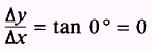Integrated Publishing, Inc. - A (SDVOSB) Service Disabled Veteran Owned Small Business